### Total 39 Videos found in Category "Core - Mathematics - III"

Title
 1Mod-1 Lec-1 Solution of ODE of First Order and First Degree 2Mod-1 Lec-10 Applications of Laplace Transformation-I 3Mod-1 Lec-11 Applications of Laplace Transformation-II 4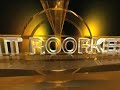Mod-1 Lec-12 One Dimensional Wave Equation 5Mod-1 Lec-13 One Dimensional Heat Equation 6Mod-1 Lec-2 Linear Differential Equations of the First Order and Orthogonal Trajectories 7Mod-1 Lec-3 Approximate Solution of An Initial Value 8Mod-1 Lec-4 Series Solution of Homogeneous Linear Differential Equations-I 9Mod-1 Lec-5 Series Solution of Homogeneous Linear Differential Equations-II 10Mod-1 Lec-6 Bessel Functions and Their Properties-I 11Mod-1 Lec-7 Bessel Functions And Their Properties-II 12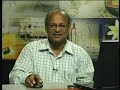Mod-1 Lec-8 Laplace Transformation-I 13Mod-1 Lec-9 Laplace Transformation-II 14Mod-2 Lec-1 Introduction to Differential Equation 15Mod-2 Lec-10 Strum Liouville boundary Value Problme 16Mod-2 Lec-11 Fourier Series-Part-1 17Mod-2 Lec-12 Fourier Series-Part-2 18Mod-2 Lec-13 Convergence of the Fourier Series 19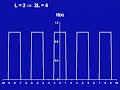Mod-2 Lec-14 Fourier Integrals 20Mod-2 Lec-15 Fourier Transforms 21Mod-2 Lec-16 Partial Differential Equation 22Mod-2 Lec-17 First Order Partial Differential Equation 23Mod-2 Lec-18 Second Order Partial Differential Equations-I 24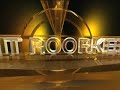Mod-2 Lec-19 Second Order Partial Differential Equations-II 25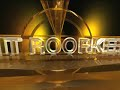Mod-2 Lec-2 First Order Differential Equations and Their Geometric Interpretation 26Mod-2 Lec-20 Solution of One Dimensional Wave Equation 27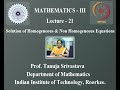Mod-2 Lec-21 Solution of Homogeneous&Non Homogeneous Equations 28Mod-2 Lec-22 Fourier Integral&Transform Method for Heat Equation 29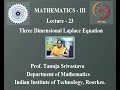Mod-2 Lec-23 Three Dimensional Laplace Equation 30Mod-2 Lec-24 Solution of Drichlet Problem 31Mod-2 Lec-25 Numerical Method for Laplace Poisson Eqaution 32Mod-2 Lec-26 ADI Method for Laplace and Poisson Equation 33Mod-2 Lec-3 Differential Equations of First Order&Higher Degree 34Mod-2 Lec-4 Linear Differential Equation of Second Order-Part-1 35Mod-2 Lec-5 Linear Differential equation of Second Order-Part-2 36Mod-2 Lec-6 Euler-Cauchy Theorem 37Mod-2 Lec-7 Higher Order Linear Differential Equations 38Mod-2 Lec-8 Higher Order Non Homogeneous Linear Equations 39Mod-2 Lec-9 Boundary Value Problems

Say and share some thing about these videos...